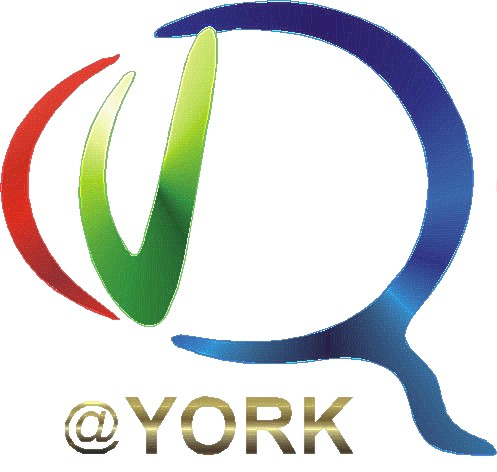"MATLAB 101"

AN INTRODUCTION TO PROGRAMMING WITH MATLAB

(a short web-course by Ricardo Tabone, Matlab programmer for JD Crawford's Lab)

 LESSON EXCERCISES ANSWERS Lesson 1 - Introduction Excercises 1 Answers 1 Lesson 2 - Matrix Operations Excercies 2 Answers 2 Lesson 3 - For Loop and Graphics Excercies 3 Answers 3